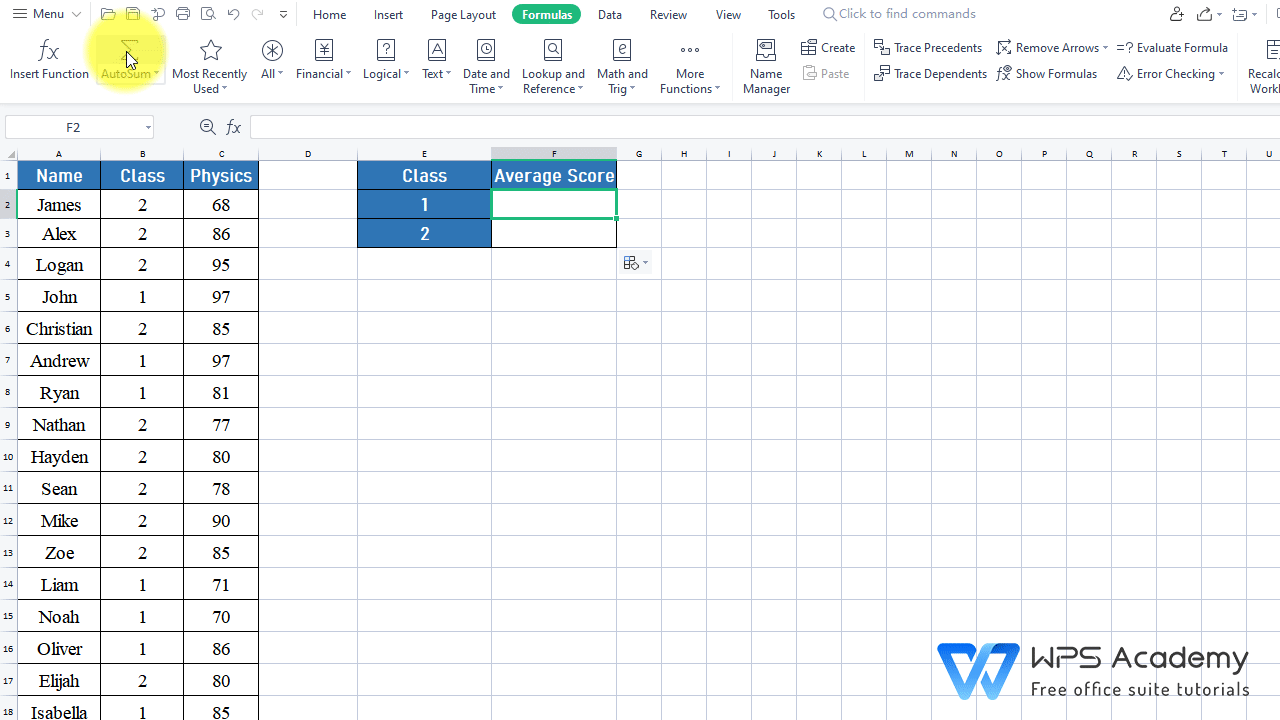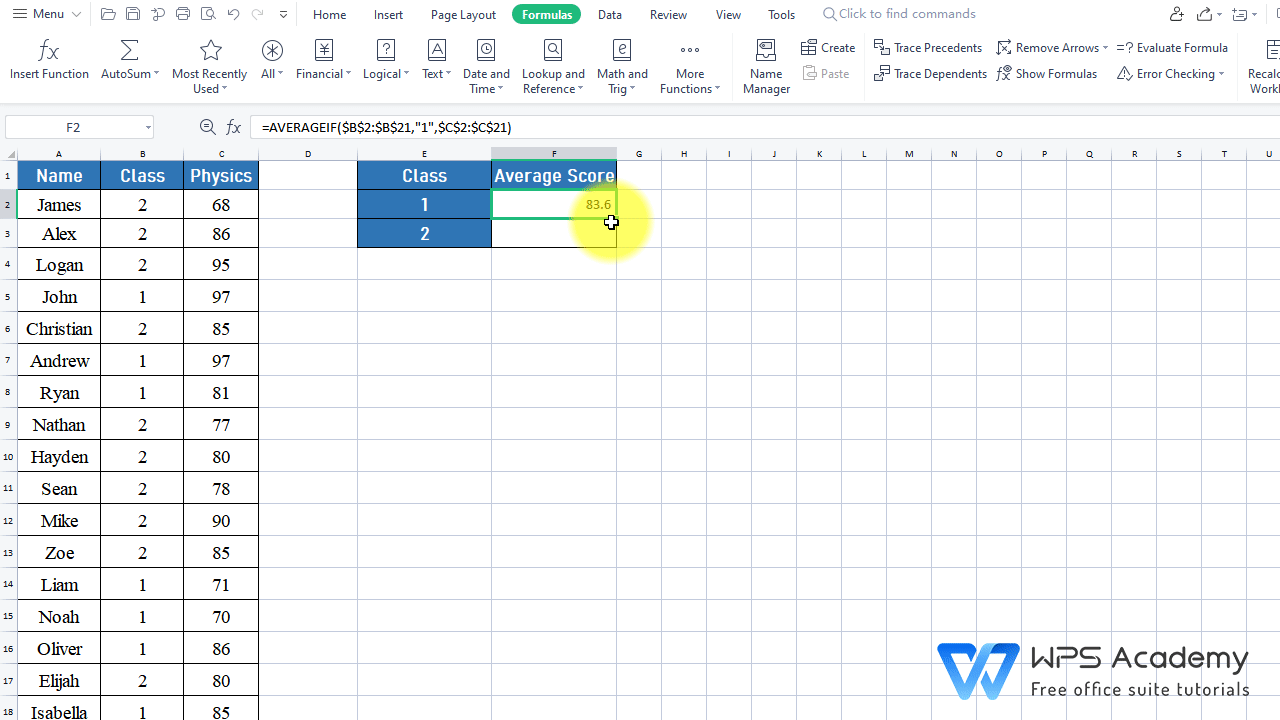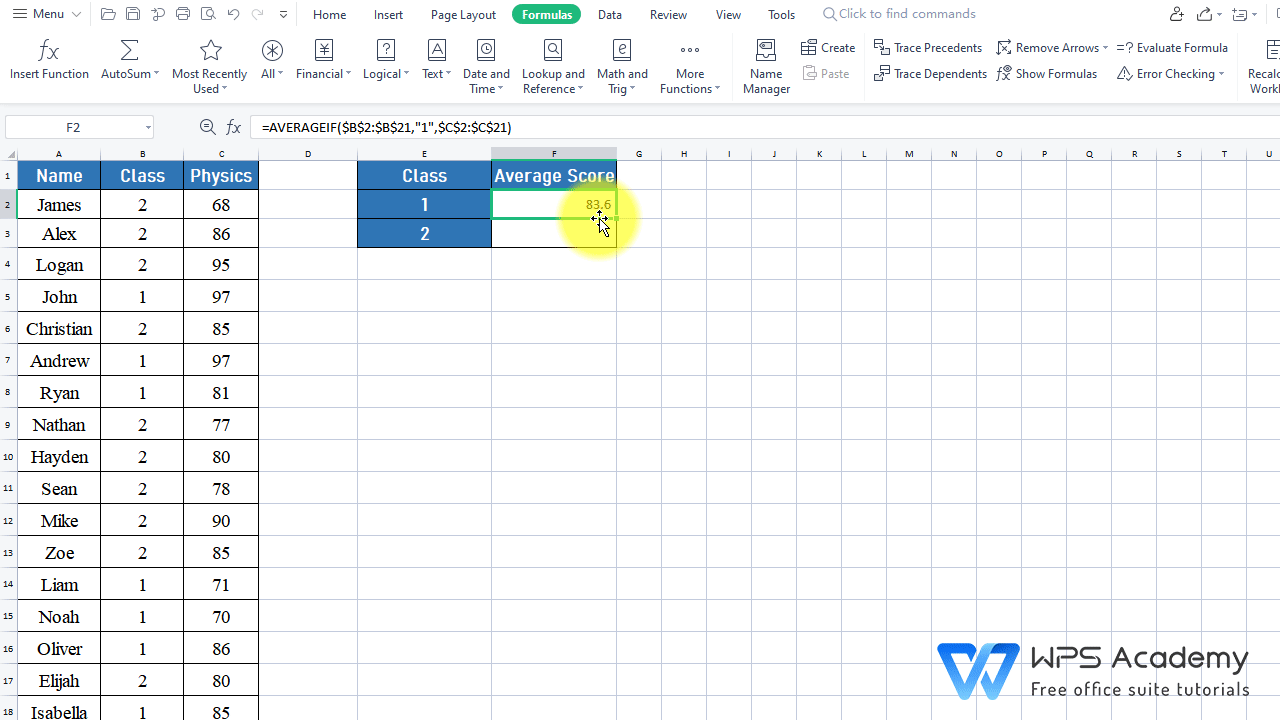The AVERAGEIF Function in Excel

December 31, 2021
82 Views

Graphic skills

WPS Spreadsheet could be an alternative to Microsoft Office Excel. It includes 100's of built-in formulas, pivot tables, and more.

· Description:

The AVERAGEIF function can finds average (arithmetic mean) for the cells specified by a given condition or criteria.

· Syntax:

AVERAGEIF(range, criteria, average_range)

· Arguments:

Range: The range of cells you want evaluated

Criteria: The condition or criteria in the form of a number, expression, or text that defines which cells will be used to find the average.

Average_range: The actual cells to be used to find the average. If omitted, cells in the range will be used.

· Example:

Assume that we want to find the average score for physics in Class 1 and Class 2 respectively.

2. Head to the Formulas tab > Insert Function > enter AVERAGEIF in the edit box.

Here we want to insert the AVERAGEIF function.

1)Range:  The range of cells you want evaluated. B2:B21 is the area we want to look up, so we enter B2:B21 at Range. We need to press F4 to make them become absolute cell so that when we pull down, the selected area won't change.

2)Criteria: The condition or criteria that defines which cells will be used to find the average. 1 stands for class 1, the criteria we set. So we enter 1 at Criteria.

3)Average_range: The actual cells to be used to find the average. If omitted, cells in the range will be used. C2:C21 is the area that contains the cells to average. So we put C2:C21 here. Similarly, we need to press F4 to make them become absolute cell so that when we pull down, the selected area won't change.

In the pop-up box, enter B2:B21 at Range, 1 at Criteria and C2:C21 at Average_range, and press F4 to both B2:B21 and C2:C21.

Finally, we can get the average score of class 1 is 83.6.3. Drag down cell F2 to cell F3 to update the data, we need to change 1 to 2. Also, we can replace 1 with E2.

(Compared with the absolute cell that we have locked in step3, E2 is a relative reference that will update itself when being dragged. )4. Finally, we can get the average score for class 1 is 83.6 while that for class 2 is 82.9.